# numpy中一些用法汇总

import numpy as np

1. 奇异值分解会用到

numpy.linalg.svd(afull_matrices=Truecompute_uv=True)

a : 维度大于等于2的矩阵

full_matrices： 默认为True ,用来表示u, vh是否维度分别为（M,M）和（N,N），不然的话维度就为（M,K）和（K,N）

compute_uv:  默认为True，是否在s外组合u和vh

u : 是个（M,M）或者（M,K）的矩阵

s ：返回的是除了主对角线以外全为0，主对角线上的值被称为奇异值，这里返回的时候只返回奇异值数组，而且奇异值是从大到小排序的，这里在做奇异值能量占比的时候会使用到。

vh ： 是个（N,N）或者（K,N）的矩阵

,    所以上述的函数就是将一个矩阵进行分解，但是在实际的操作中是要将矩阵进行降维，降维主要是对中间的 进行调整，这是个对角矩阵，因为除了主对角线以外其余的元素全为0，而在做奇异值分解后实际返回的奇异值大小是从大往小排序的，我们在实际操作中只取前面的k个值，前10%的奇异值之和就占了全部奇异值之和的80%以上的比例，从而达到降维的效果。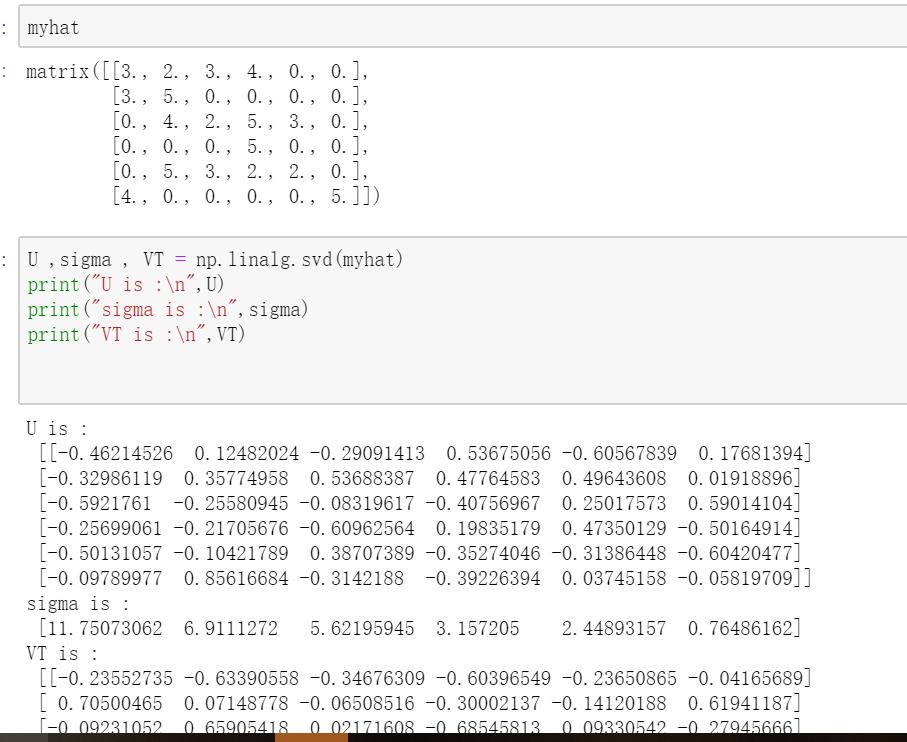比如上述的sigma我们可以看到奇异值是从大往小排序的，前5就能包含89%的特征了，那么我们取5*5来对矩阵进行降维后看会得到啥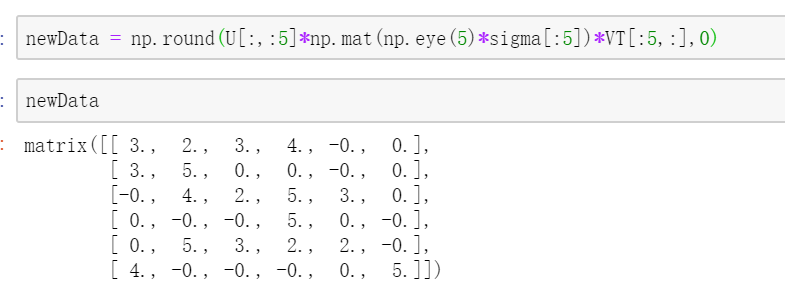2. 保留小数位

a: array 或者 矩阵

decimals ： 精度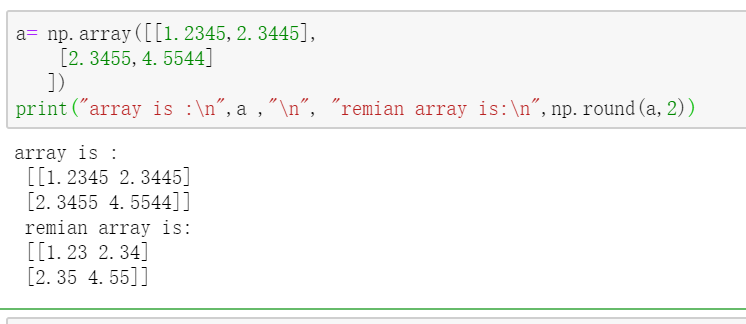3. 矩阵的叉乘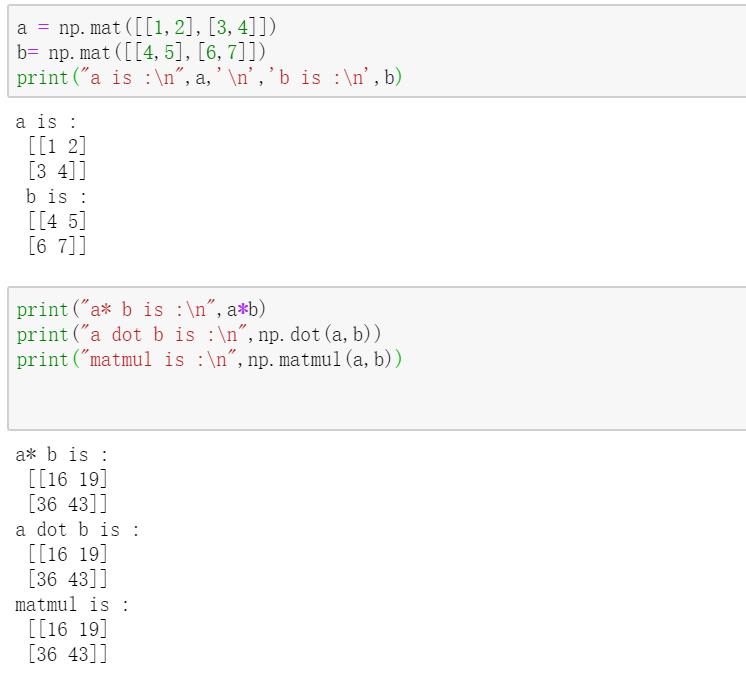4. 矩阵的模 numpy.linalg.norm(xord=Noneaxis=Nonekeepdims=False)

x: array 或者矩阵

ord ： 默认为None，范式 的顺序，比如向量的1-范式：向量元素绝对值之和，向量的2-范式 ：欧几里得范数，常用计算向量长度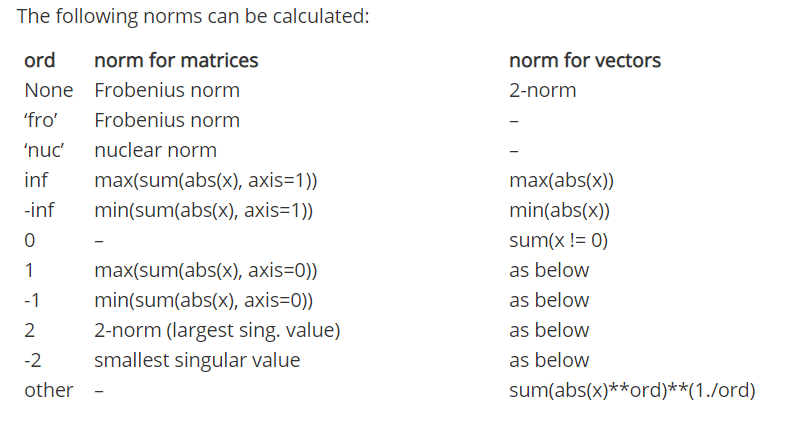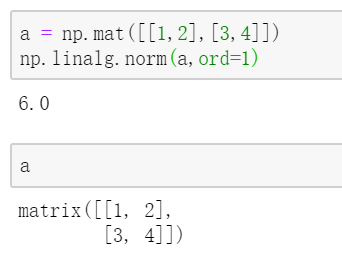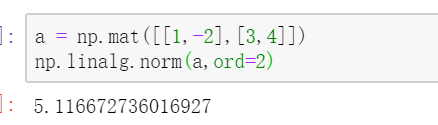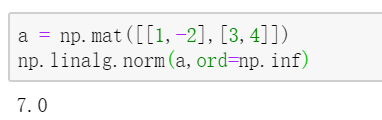5. numpy.sum() ，如果使用了np.sum ，那么表示将一个数组中的维数和列数上的数都加在一起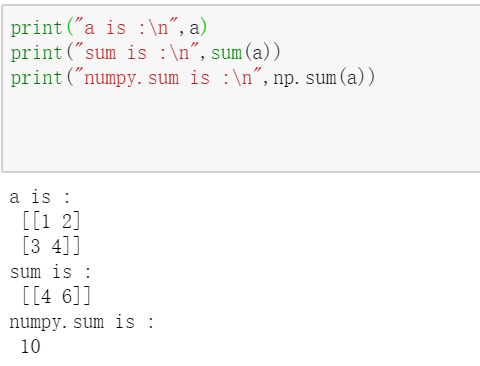6.  numpy.reshape(a,newshape,order='C')  矩阵重组，不改变矩阵内的数据，只改变维度

a: 目标矩阵或者array

newshape: 新维度，整型或者为整型的元组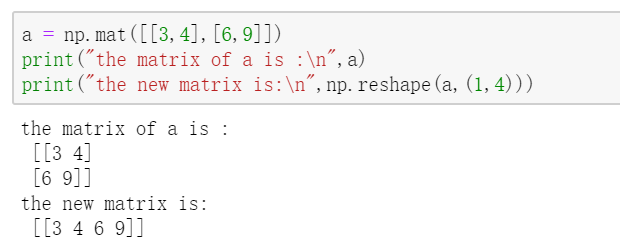import numpy as np
x = np.array([0,1,2,3,3,4,5])
print(x.reshape(1, -1))
print(x.reshape(1, 7))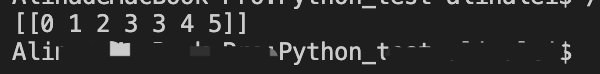print(x.reshape(-1, 1))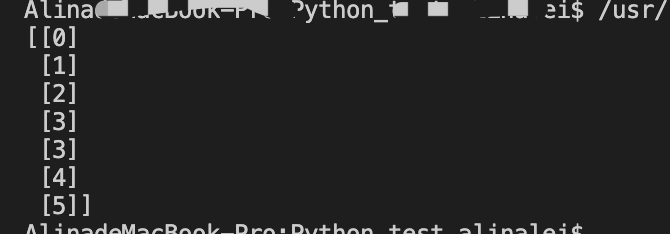7.  矩阵的合并

numpy.vstack((a,b))  、numpy.hstack((a,b))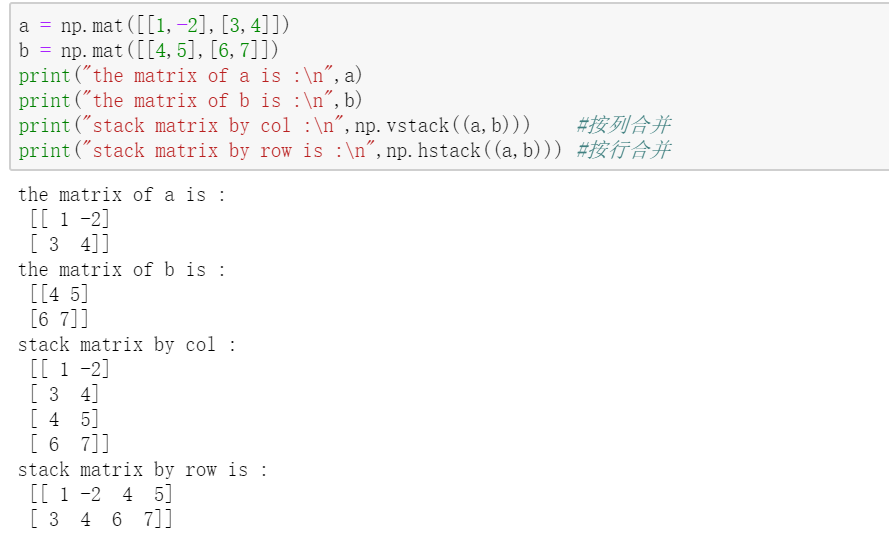8. 矩阵的列和和行和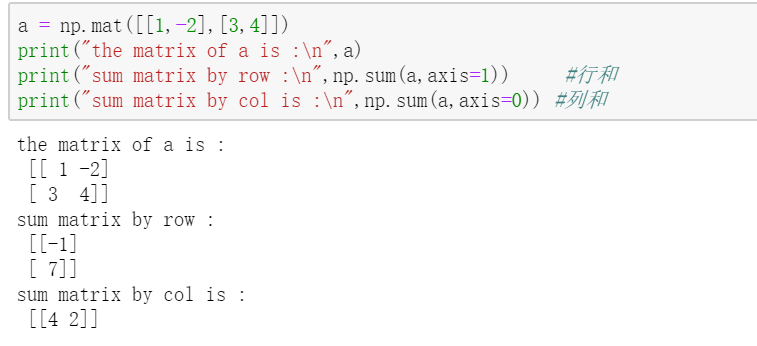9.   生成对角矩阵

numpy.eye(n,n)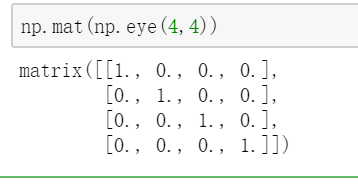10.生成一个对角线为按照a列表来的矩阵

numpy.diag(a)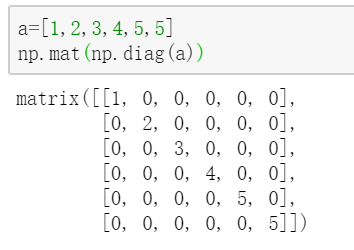11. 矩阵的逆矩阵和转置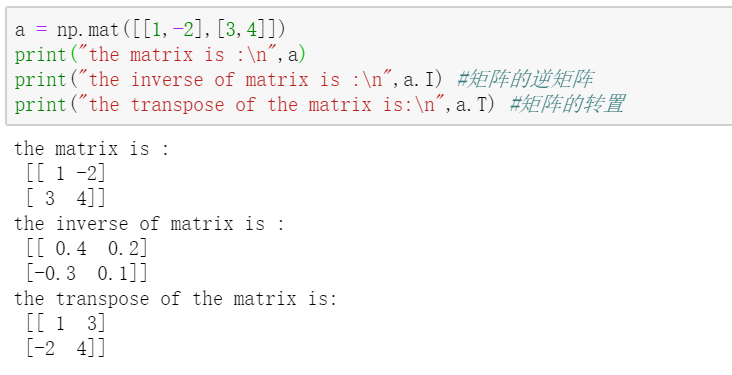12 .矩阵的特征值 、特征向量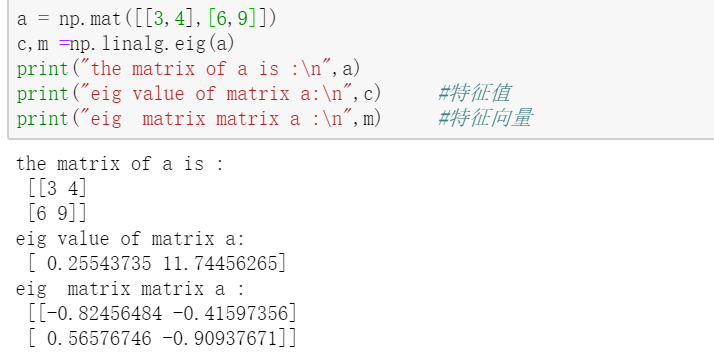13. 返回非0元素的索引值

numpy.nonzero(a)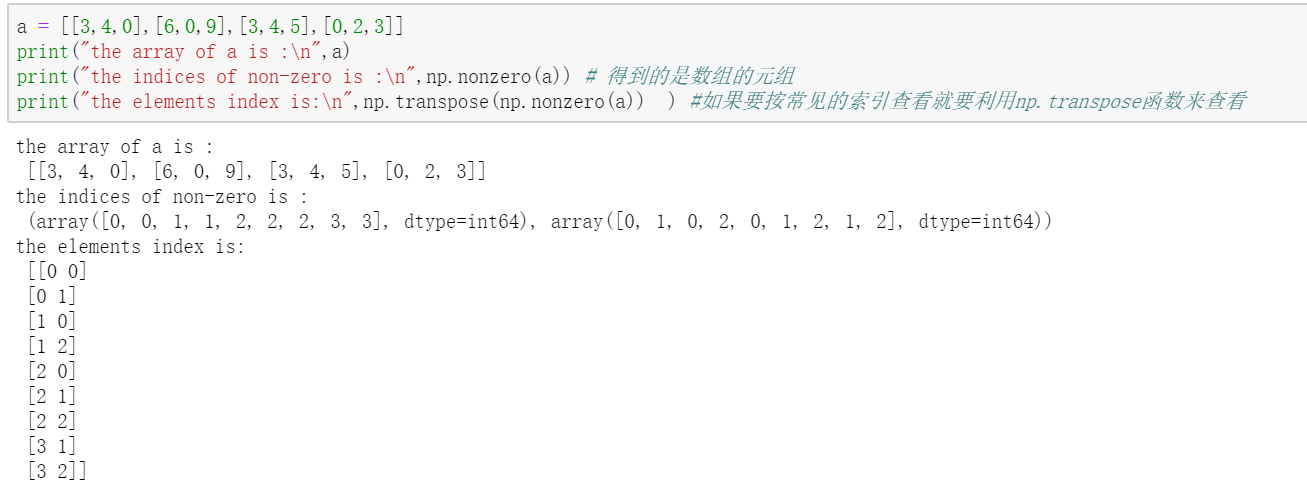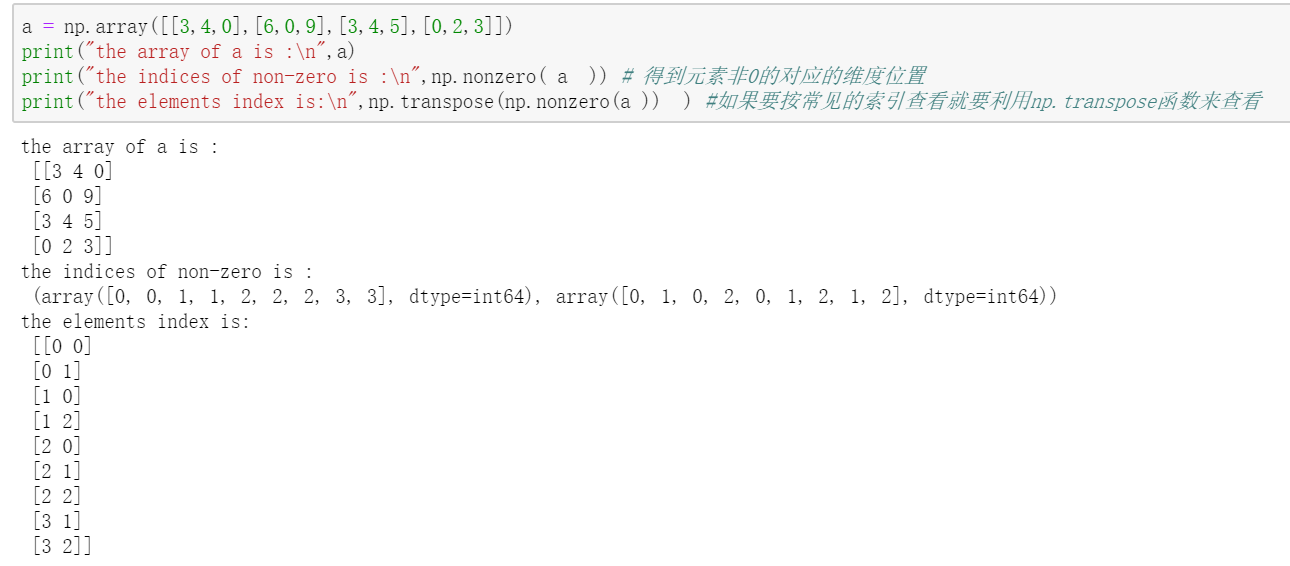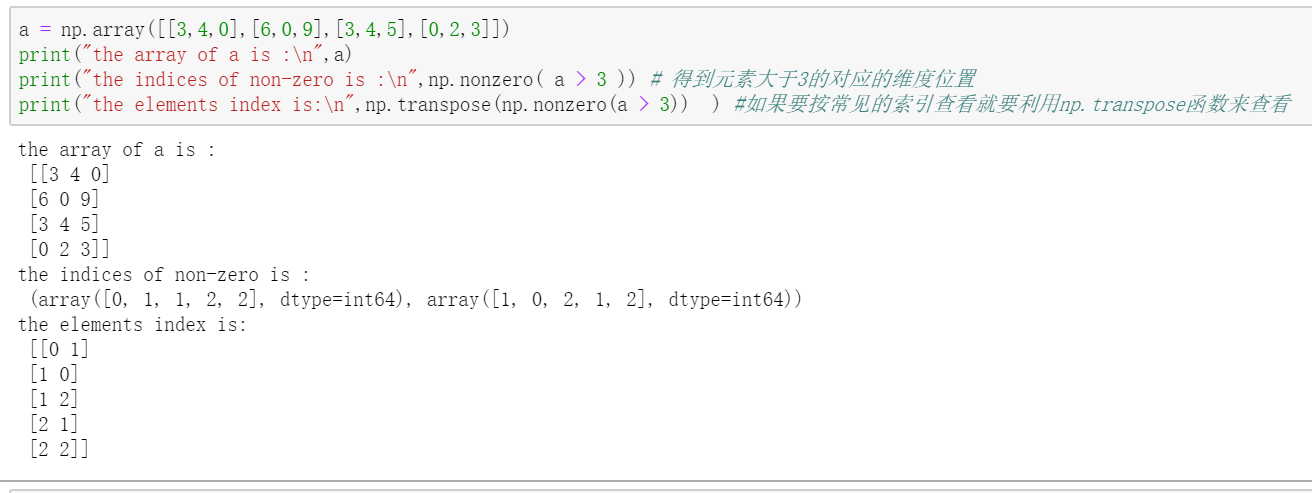14. 数组拼接 numpy.concatenate(array, axis=0)

array 待拼接数组列表

axis 为0 代表将多个待拼接数组进行行追加对行扩充，为1代表将多个待拼接数据进行列追加对列扩充。

a = np.array([2, 3, 4, 5])
b = np.concatenate((np.array([-float('inf')]), a, np.array([float('inf')])))
print('b is:', b)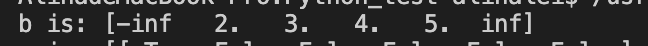15. numpy.where(condition, x, y)

07-012万+04-015123
06-09317
12-027635
06-24122
10-263204
01-08212
09-1714
08-12371